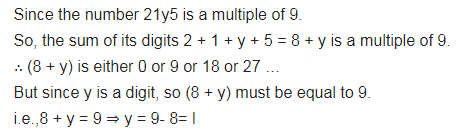# If 21 y 5 is a multiple of 9, where y is a digit, what is the value of y?

If 21 y 5 is a multiple of 9, where y is a digit, what is the value of y?